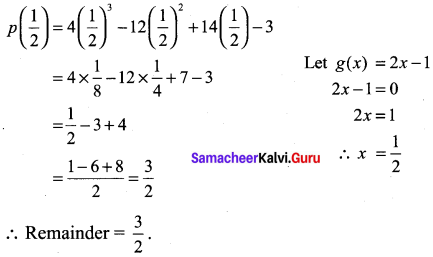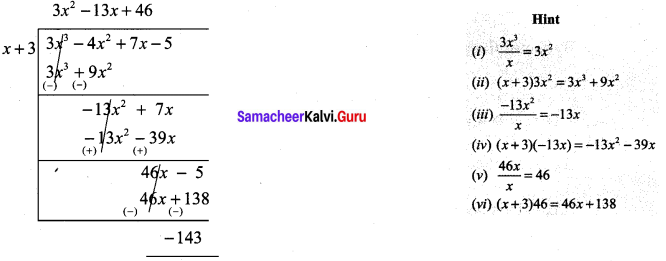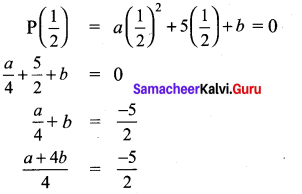## Tamilnadu Samacheer Kalvi 9th Maths Solutions Chapter 3 Algebra Ex 3.3

9th Maths Algebra Exercise 3.3 Question 1.
Check whether p(x) is a multiple of g(x) or not.
p(x) = x3 – 5x2 + 4x – 3, g(x) = x – 2
Solution:
p(x) = x3 – 5x2 + 4x – 3; g(x) = x – 2
Let g(x) = 0
x – 2 = 0
x = 2
p(2) = 23 – 5(22) + 4(2) – 3
= 8 – 5 × 4 + 8 – 3 = 8 – 20 + 5 = -7 ≠ 0
⇒ p(x) is not a multiple of g(x)

9th Maths Exercise 3.3 Question 2.
By remainder theorem, find the remainder when, p(x) is divided by g(x) where,
(i) p(x) = x3 – 2x2 – 4x – 1; g(x) = x + 1
(ii) p(x) = 4x3 – 12x2 + 14x – 3; g(x) = 2x – 1
(iii) p(x) = x3 – 3x2 + 4x + 50; g(x) = x – 3
Solution:
(i) p(x) = x3 – 2x2 – 4x – 1; g(x) = x + 1
Let g(x) = x + 1
x + 1 = 0
x = -1
P(-1) = (-1)3 – 2(-1)2 – 4(-1) – 1
= -1 -2 × 1 + 4 – 1
= -4 + 4 = 0
∴ Remainder = 0.

(ii) p(x) = 4x3 – 12x2 + 14x – 3; g(x) = 2x – 1(iii) p(x) = x3 – 3x2 + 4x + 50 ; g(x) = x – 3
Let g(x) = x – 3
x – 3 = 0
x = 3
p(3) = 33 – 3(32) + 4(3) + 50
= 27 – 27 + 12 + 50
= 62
∴ Remainder = 62.

9th Maths 3.3 Question 3.
Find the remainder when 3x3 – 4x2 + 7x – 5 is divided by (x + 3)
Solution:
(3x3 – 4x2 + 7x – 5) + (x + 3)The remainder is -143.

9th Class Maths Exercise 3.3 Solution Question 4.
What is the remainder when x2018 + 2018 is divided by x – 1.
Solution:
x2018 + 2018 is divided by x – 1
Let g(x) = x – 1 = 0
x = 1
p(x) = x2018 + 2018
p(1)= 12018 + 2018
= 1 + 2018 = 2019

Samacheer Kalvi 9th Maths Chapter 3 Question 5.
For what value of k is the polynomial p(x) = 2x3 – kx2 + 3x + 10 exactly divisible by (x – 2).
Solution:
Let g(x) = x – 2 = 0
x = 2
Since p(x) is exactly divisible by (x – 2)
p(2) = 2(23) – k(22) + 3(2)+ 10
= 16 – 4k + 6 + 10
= 32 – 4k = 0
= -k = -32
k= $$\frac{32}{4}$$ = 8.

Ex 3.3 Class 9 Samacheer Question 6.
If two polynomials 2x3 + ax2 + 4x – 12 and x3 + x2 – 2x + a leave the same remainder when divided by (x – 3), find the value of a. and also find the remainder.
Solution:
Let f(x) = 2x3 + ax2 + 4x – 12 and g(x) = x3 + x2 – 2x + a
When f(x) is divided by x – 3, the remainder is f(3).
Now f(3) = 2(3)3 + a(3)2 + 4(3) – 12 = 54 + 9a + 12 – 12
f(3) = 9a + 54 …………. (1)
When g(x) is divided by x – 3, the remainder is g(3).
Now g(3) = 33 + 32 – 2(3) + a = 27 + 9 – 6 + a
g(3) = a + 30 ……….. (2)
Since, the remainder’s are same (1) = (2)
Given that f(3) = g(3)
That is 9a + 54 = a + 30
9a – a = 30 – 54 ⇒ 8a = -24 ∴ a = -3
Substituting a = -3 in f(3), we get
f(3) = 9(-3) + 54 = -27 + 54
f(3) = 27
∴ The remainder is 27.

9th Class Math 3.3 Exercise Solution Question 7.
Determine whether (x – 1) is a factor of the following polynomials:
(i) x3 + 5x2 – 10x + 4
(ii) x4 + 5x2 – 5x + 1
Solution:
(i) Let P(x) = x3 + 5x2 – 10x + 4
By factor theorem (x – 1) is a factor of P(x), if P(1) = 0
P(1) = 13 + 5(12) – 10(1) + 4 = 1 + 5 – 10 + 4
P(1) = 0
∴ (x – 1) is a factor of x3 + 5x2 – 10x + 4

(ii) Let P(x) = x4 + 5x2 – 5x + 1
By/actor theorem, (x – 1) is a factor of P(x), if P( 1) = 0
P(1) = 14 + 5 (12) – 5(1) + 1 = 1 + 5 – 5 + 1 = 2 ≠ 0
∴ (x – 1) is not a factor of x4 + 5x2 – 5x + 1

Class 9th Maths Exercise 3.3 Question 8.
Using factor theorem, show that (x – 5) is a factor of the polynomial 2x3 – 5x2 – 28x + 15
Solution:
LetP(x) = 2x3 – 5x2 – 28x + 15
By factor theorem, (x – 5) is a factor of P(x), if P(5) = 0
P(5) = 2 (5)2 – 5 (5)2 – 28 (5) + 15
= 2 × 125 – 5 × 25 – 140 + 15
= 250 – 125 – 140 + 15 = 265 – 265 = 0
∴ (x – 5) is a factor of 2x3 – 5x2 – 28x + 15

Class 9 Maths Exercise 3.3 Solutions Question 9.
Determine the value of m, if (x + 3) is a factor of x3 – 3x2 – mx + 24.
Solution:
Let P(x) = x3 – 3x2 – mx + 24
By using factor theorem,
(x + 3) is a factor of P(x), then P (-3) = 0
P(-3) = (-3)3 -3 (-3)2 – m (-3) + 24 = 0
⇒ -27 – 3 × 9 + 3m + 24 = 0 ⇒ 3m = 54 – 24
⇒ m = $$\frac{30}{3}$$ = 10

Exercise 3.3 Class 9 Maths Question 10.
If both (x – 2) and (x – $$\frac{1}{2}$$) are the factors of ax2 + 5x + b, then show that a = b.
Solution:
Let P(x) = ax2 + 5x + b
(x – 2) is a factor of P(x), if P(2) = 0
P(2) = a(2)2 + 5(2) + b = 0
4a + 10 + b = 0
4 a + b = – 10 …………….. (1)
(x – $$\frac{1}{2}$$) is a factor of P(x), P($$\frac{1}{2}$$) = 02a + 8b = -20
a + 4b = – 10 ……………… (2)
From (1) and (2)
4a + b = – 10 …………. (1)
a + 4b = – 10 ………… (2)
(1) and (2) ⇒ 4a + b = a + 4b
3a = 3b
∴ a = b. Hence it is proved.

Ex 3.3 Class 9 Maths Solutions Question 11.
If (x – 1)divides the polynomial kx3 – 2x2 + 25x – 26 without remainder, then find the value of k.
Solution:
Let P(x) = kx3 – 2x2 + 25x – 26
By factor theorem, (x – 1) divides P(x) without remainder, P (1) = 0
P(1) = k(1)3 -2 (1)2 + 25 (1) – 26 = 0
k – 2 + 25 – 26 = 0
k – 3 =0
k = 3

Class 9th Math Exercise 3.3 Question 12.
Check if (x + 2) and (x – 4) are the sides of a rectangle whose area is x2 – 2x – 8 by using factor theorem.
Solution:
Let P(x) = x2 – 2x2 – 8
By using factor theorem,(x + 2) is a factor of P(x), if P (-2) = 0
P(-2) = (-2)2 – 2 (-2) – 8 = 4 + 4 – 8 = 0
and also (x -4) is a factor of P (x), if P (4) = 0
p (4) = 42 – 2(4) – 8 = 16 – 8 – 8 = 0
∴ (x + 2), (x – 4) are the sides of a rectangle whose area is x2 – 2x – 8.